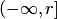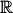# P-value

Conformal predictors can be packaged as conformal transductors, which output p-values. As statisticians often emphasize, p-values are not probabilities. A small p-value means that a statistical hypothesis can be rejected; therefore, what matters for p-values is whether they are small or not, not their precise magnitude.

The method of Venn prediction is similar to conformal prediction but Venn predictors output probabilities rather than p-values. It is interesting that in some cases p-values coalesce to form predictive distributions, in which case they acquire properties of probabilities, as in conformal predictive systems. (In particular, their precise magnitudes become important.)

See Gurevich and Vovk (2017) for an in-depth study of p-values. One of the findings of this paper is that test statistics that take values in arbitrary linearly ordered sets (with measurable initial segments) do not always produce valid p-values; imposing the restriction that the linear order is short resolves this difficulty (practical statisticians usually consider test statistics that take values in the real line, which is short, and therefore, they are on safe ground). However, this does not mean that we cannot generalize the notions of conformal predictor and conformal transducer to conformity measures taking values in an arbitrary linearly ordered set rather than; in fact, it is easy to see that the standard properties of validity remain intact without the shortness requirement.

Bibliography Yuri Gurevich and Vladimir Vovk (2017). Test statistics and p-values. On-line Compression Modelling Project (New Series), Working Paper 16.